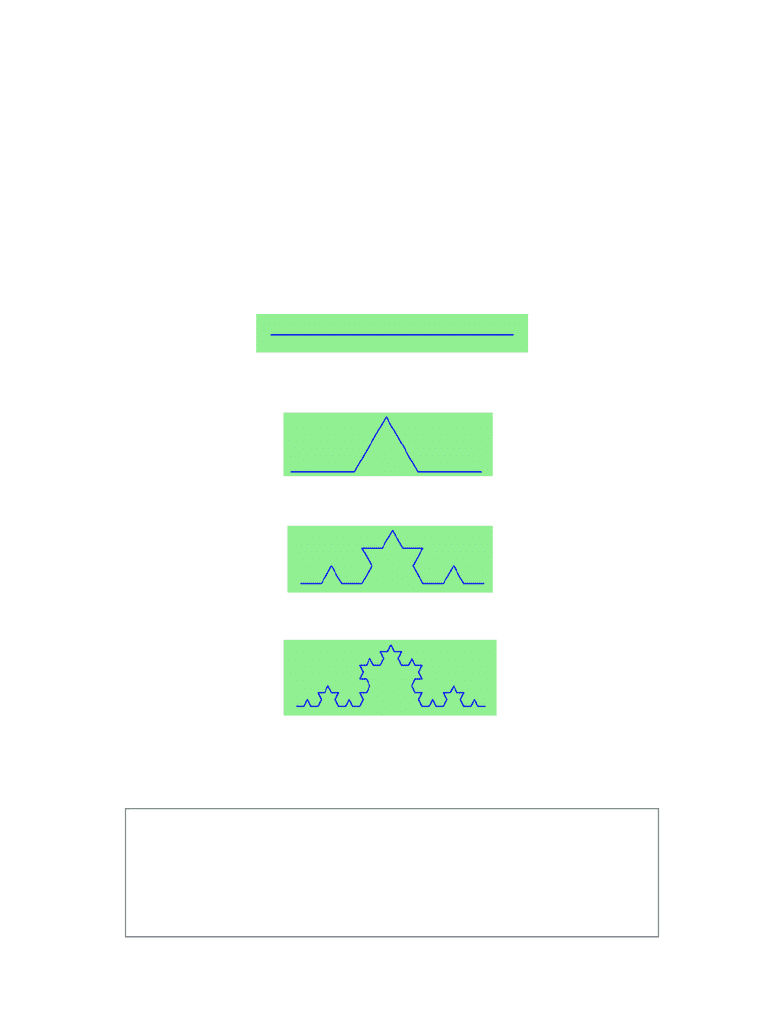# CSCA48H3 Lecture Notes - Lecture 4: Init, Operand, Alien 3

76 views7 pages
School
CourseCSC A48 — Intro to Computer Science II
Winter 2016
Chapter 3: Recursion
Recursion means “defining something in terms of itself” usually at some smaller scale, perhaps multiple
times, to achieve your objective. For example, we might say “A human being is someone whose mother is
a human being”, or “a directory is a structure that holds files and (smaller) directories”, or “a family tree
starts with a couple who have children, each with their own family sub-trees”.
Programming languages generally support recursion, which means that, in order to solve a problem,
functions can call themselves to solve smaller subproblems.
1. Drawing Fractals
For our purposes, a fractal is a drawing which also has self-similar structure, where it can be defined in
terms of itself.
Let us start by looking at the famous Koch fractal. An order 0 Koch fractal is simply a straight line of a
given size.
An order 1 Koch fractal is obtained like this: instead of drawing just one line, draw instead four smaller
segments, in the pattern shown here:
Now what would happen if we repeated this Koch pattern again on each of the order 1 segments? We’d get
this order 2 Koch fractal:
Repeating our pattern again gets us an order 3 Koch fractal:
Now let us think about it the other way around. To draw a Koch fractal of order 3, we can simply draw
four order 2 Koch fractals. But each of these in turn needs four order 1 Koch fractals, and each of those in
turn needs four order 0 fractals. Ultimately, the only drawing that will take place is at order 0. This is very
simple to code up in Python:
Page ! of !1 7
def koch(t, order, size):
"""
Make turtle t draw a Koch fractal of 'order' and 'size'.
Leave the turtle facing the same direction.
"""
if order == 0: # The base case is just a straight line
t.forward(size)
Unlock document

This preview shows pages 1-2 of the document.
Unlock all 7 pages and 3 million more documents.

Already have an account? Log inCSC A48 — Intro to Computer Science II
Winter 2016
The key thing that is new here is that if order is not zero, koch calls itself recursively to get its job done.
Let’s make a simple observation and tighten up this code. Remember that turning right by 120 is the same
as turning left by -120. So with a bit of clever rearrangement, we can use a loop instead of lines 10-16:
The final turn is 0 degrees — so it has no effect. But it has allowed us to find a pattern and reduce seven
lines of code to three, which will make things easier for our next observations.
Page ! of !2 7
else:
koch(t, order-1, size/3) # Go 1/3 of the way
t.left(60)
koch(t, order-1, size/3)
t.right(120)
koch(t, order-1, size/3)
t.left(60)
koch(t, order-1, size/3)
def koch(t, order, size):
if order == 0:
t.forward(size)
else:
for angle in [60, -120, 60, 0]:
koch(t, order-1, size/3)
Recursion, the high-level view
——————————————————————————————————————————————
One way to think about this is to convince yourself that the function works correctly when you call
it for an order 0 fractal. Then do a mental leap of faith, saying “the fairy godmother (or Python, if
you can think of Python as your fairy godmother) knows how to handle the recursive level 0 calls
for me on lines 11, 13, 15, and 17, so I don’t need to think about that detail!” All I need to focus on
is how to draw an order 1 fractal if I can assume the order 0 one is already working.
You’re practicing mental abstraction — ignoring the subproblem while you solve the big problem.
If this mode of thinking works (and you should practice it!), then take it to the next level. Aha!
now can I see that it will work when called for order 2 under the assumption that it is already
working for level 1.
And, in general, if I can assume the order n-1 case works, can I just solve the level n problem?
Students of mathematics who have played with proofs of induction should see some very strong
similarities here.
Recursion, the low-level operational view
——————————————————————————————————————————————
Another way of trying to understand recursion is to get rid of it! If we had separate functions to
draw a level 3 fractal, a level 2 fractal, a level 1 fractal and a level 0 fractal, we could simplify the
above code, quite mechanically, to a situation where there was no longer any recursion, like this:
def koch_0(t, size):
t.forward(size)
def koch_1(t, size):
for angle in [60, -120, 60, 0]:
koch_0(t, size/3)
t.left(angle)
Unlock document

This preview shows pages 1-2 of the document.
Unlock all 7 pages and 3 million more documents.

Already have an account? Log in

# Get access

Grade+
\$10 USD/m
Billed \$120 USD annually
Homework Help
Class Notes
Textbook Notes
40 Verified Answers
Study Guides
1 Booster Class
Class+
\$8 USD/m
Billed \$96 USD annually
Homework Help
Class Notes
Textbook Notes
30 Verified Answers
Study Guides
1 Booster Class